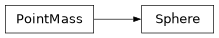# geoana.gravity.Sphere#Class for gravitational solutions for a sphere.

The Sphere class is used to analytically compute the gravitational potentials, fields, and gradients for a sphere.

Parameters:
rhofloat

Density of sphere ($$\frac{kg}{m^3}$$).

massfloat

Mass of the sphere (kg). Default is $$m = \frac{4}{3} \pi R^3 \rho$$ kg.

locationarray_like, optional

Center of the sphere (m). Default is (0, 0, 0).

Attributes

 location Location of the point mass. mass Mass of sphere in kilograms. radius Radius of the sphere in meters. rho Density of the sphere in kilograms over meters cubed.

Methods

 Gravitational field due to a sphere. Gravitational gradient for a sphere. Gravitational potential due to a sphere.